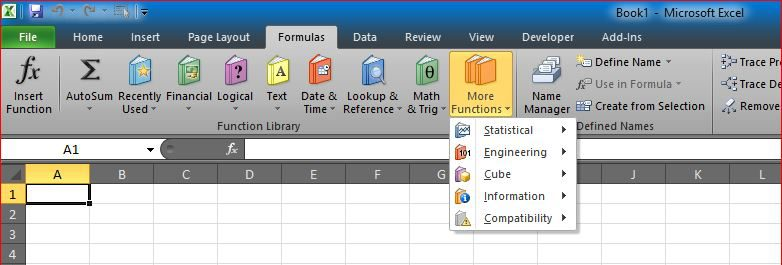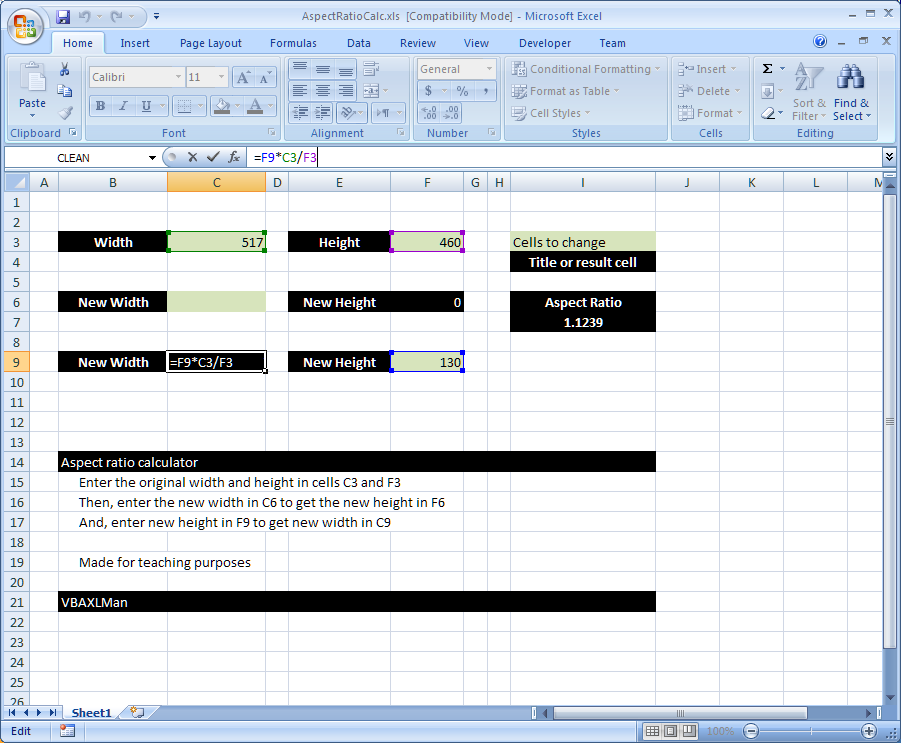# Standard math to calculate Aspect RatioHere is a simple formula that uses standard math functions (+ , – , / and *) to calculate the aspect ratio

I often need some simple formula like this to get image dimensions

Two formulas used here, one in C9:

`=F9*C3/F3`

And another one in cell F7:

`=C6*F3/C3`